## codeigniter里pgsql驱动的一些问题

为什么80%的码农都做不了架构师？->>>Codeigniter的pgsql驱动是不是不太完整的，昨天遇到了一个问题，就是查询一些记录的时候使用了时间进行DESC排序，但结果是完全没有生效，然后我直接用id进行DESC排序，它就可以排了。

0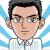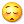我知道是什么原因了！！

modified 字段被截断了了。所以没有效果。

codeigniter 是访问pgsql是没有问题的。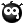0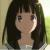许多PHP框架里对其他数据库的支持都是狗尾续貂的摆设而已，因为开发者都没其它数据库的深度使用经验，里面的支持仅仅是按照ANSI SQL的基本语法实现的，大多没考虑其它数据库的特性。

CI对PG不存在什么驱动支持，和PHP自身提供的PG驱动也不是一个层次，CI对PG的支持就是写了个类，使用了PHP提供的PG_函数而已。不要拌浆糊混作一谈。如果PHP不支持，那CI自然不支持。

不过看过你的描述，看起来更像是你代码的问题。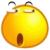0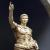0Punbb 里面的一个类, 修改过的:

```<?php
/**
* A database layer class that relies on the PostgreSQL PHP extension.
*
* @copyright (C) 2008-2009 PunBB, partially based on code (C) 2008-2009 FluxBB.org
* @license http://www.gnu.org/licenses/gpl.html GPL version 2 or higher
* @package PunBB
*/

// Make sure we have built in support for PostgreSQL
if (!function_exists('pg_connect'))
exit('This PHP environment doesn\'t have PostgreSQL support built in. PostgreSQL support is required if you want to use a PostgreSQL database to run this forum. Consult the PHP documentation for further assistance.');

class DBLayer
{
var \$prefix;
var \$query_result;
//var \$last_query_text = array();
var \$in_transaction = 0;

var \$saved_queries = array();
var \$num_queries = 0;

var \$error_no = false;
var \$error_msg = 'Unknown';

var \$datatype_transformations = array(
'/^(TINY|SMALL)INT( )?(\\([0-9]+\\))?( )?(UNSIGNED)?\$/i'			=>	'SMALLINT',
'/^(MEDIUM)?INT( )?(\\([0-9]+\\))?( )?(UNSIGNED)?\$/i'				=>	'INTEGER',
'/^BIGINT( )?(\\([0-9]+\\))?( )?(UNSIGNED)?\$/i'						=>	'BIGINT',
'/^(TINY|MEDIUM|LONG)?TEXT\$/i'										=>	'TEXT',
'/^DOUBLE( )?(\\([0-9,]+\\))?( )?(UNSIGNED)?\$/i'					=>	'DOUBLE PRECISION',
'/^FLOAT( )?(\\([0-9]+\\))?( )?(UNSIGNED)?\$/i'						=>	'REAL'
);

function __construct (\$db_host, \$db_username, \$db_password, \$db_name, \$db_prefix, \$p_connect)
{
\$this->prefix = \$db_prefix;

if (\$db_host)
{
if (strpos(\$db_host, ':') !== false)
{
list(\$db_host, \$dbport) = explode(':', \$db_host);
\$connect_str[] = 'host='.\$db_host.' port='.\$dbport;
}
else
\$connect_str[] = 'host='.\$db_host;
}

if (\$db_name)
\$connect_str[] = 'dbname='.\$db_name;

if (\$p_connect)
\$this->link_id = @pg_pconnect(implode(' ', \$connect_str));
else
\$this->link_id = @pg_connect(implode(' ', \$connect_str));

error('Unable to connect to PostgreSQL server.', __FILE__, __LINE__);

// Setup the client-server character set (UTF-8)
if (!defined('FORUM_NO_SET_NAMES'))
\$this->set_names('utf8');

}

function start_transaction()
{
++\$this->in_transaction;

return (@pg_query(\$this->link_id, 'BEGIN')) ? true : false;
}

function end_transaction()
{
--\$this->in_transaction;

return true;
else
{
return false;
}
}

function query(\$sql)
{
if (strlen(\$sql) > 140000)
exit('Insane query. Aborting.');

if (strrpos(\$sql, 'LIMIT') !== false)
\$sql = preg_replace('#LIMIT ([0-9]+),([ 0-9]+)#', 'LIMIT \\2 OFFSET \\1', \$sql);

if (defined('FORUM_SHOW_QUERIES'))
\$q_start = get_microtime();

if (pg_result_status(\$this->query_result) != PGSQL_FATAL_ERROR)
{
if (defined('FORUM_SHOW_QUERIES'))
\$this->saved_queries[] = array(\$sql, sprintf('%.5f', get_microtime() - \$q_start));

++\$this->num_queries;

//\$this->last_query_text[\$this->query_result] = \$sql;

return \$this->query_result;
}
else
{
if (defined('FORUM_SHOW_QUERIES'))
\$this->saved_queries[] = array(\$sql, 0);

\$this->error_msg = @pg_result_error(\$this->query_result);

if (\$this->in_transaction)

--\$this->in_transaction;

return false;
}
}

function query_build(\$query, \$return_query_string = false, \$return_insert_id_string = false)
{
\$sql = '';

if (isset(\$query['SELECT']))
{
\$sql = 'SELECT '.\$query['SELECT'].' FROM '.(isset(\$query['PARAMS']['NO_PREFIX']) ? '' : \$this->prefix).\$query['FROM'];

if (isset(\$query['JOINS']))
{
foreach (\$query['JOINS'] as \$cur_join)
\$sql .= ' '.key(\$cur_join).' '.(isset(\$query['PARAMS']['NO_PREFIX']) ? '' : \$this->prefix).current(\$cur_join).' ON '.\$cur_join['ON'];
}

if (!empty(\$query['WHERE']))
\$sql .= ' WHERE '.\$query['WHERE'];
if (!empty(\$query['GROUP BY']))
\$sql .= ' GROUP BY '.\$query['GROUP BY'];
if (!empty(\$query['HAVING']))
\$sql .= ' HAVING '.\$query['HAVING'];
if (!empty(\$query['ORDER BY']))
\$sql .= ' ORDER BY '.\$query['ORDER BY'];
if (!empty(\$query['LIMIT']))
\$sql .= ' LIMIT '.\$query['LIMIT'];
}
else if (isset(\$query['INSERT']))
{
\$sql = 'INSERT INTO '.(isset(\$query['PARAMS']['NO_PREFIX']) ? '' : \$this->prefix).\$query['INTO'];

if (!empty(\$query['INSERT']))
\$sql .= ' ('.\$query['INSERT'].')';

if (is_array(\$query['VALUES']))
{
\$new_query = \$query;
if (\$return_query_string)
{
\$query_set = array();
foreach (\$query['VALUES'] as \$cur_values)
{
\$new_query['VALUES'] = \$cur_values;
\$query_set[] = \$this->query_build(\$new_query, true);
}

\$sql = implode('; ', \$query_set);
}
else
{
\$result_set = null;
foreach (\$query['VALUES'] as \$cur_values)
{
\$new_query['VALUES'] = \$cur_values;
\$result_set = \$this->query_build(\$new_query, false);
}

return \$result_set;
}
}
else
\$sql .= ' VALUES('.\$query['VALUES'].')';

if (\$return_insert_id_string){
\$sql .= " returning ".\$return_insert_id_string;
}
}
else if (isset(\$query['UPDATE']))
{
\$query['UPDATE'] = (isset(\$query['PARAMS']['NO_PREFIX']) ? '' : \$this->prefix).\$query['UPDATE'];

\$sql = 'UPDATE '.\$query['UPDATE'].' SET '.\$query['SET'];

if (!empty(\$query['WHERE']))
\$sql .= ' WHERE '.\$query['WHERE'];
}
else if (isset(\$query['DELETE']))
{
\$sql = 'DELETE FROM '.(isset(\$query['PARAMS']['NO_PREFIX']) ? '' : \$this->prefix).\$query['DELETE'];

if (!empty(\$query['WHERE']))
\$sql .= ' WHERE '.\$query['WHERE'];
}
else if (isset(\$query['REPLACE']))
{
\$sql = 'INSERT INTO '.(isset(\$query['PARAMS']['NO_PREFIX']) ? '' : \$this->prefix).\$query['INTO'];

if (!empty(\$query['REPLACE']))
\$sql .= ' ('.\$query['REPLACE'].')';

\$sql .= ' SELECT '.\$query['VALUES'].' WHERE NOT EXISTS (SELECT 1 FROM '.(isset(\$query['PARAMS']['NO_PREFIX']) ? '' : \$this->prefix).\$query['INTO'].' WHERE '.\$query['UNIQUE'].')';
}

return (\$return_query_string) ? \$sql : \$this->query(\$sql);
}

function result(\$query_id = 0, \$row = 0, \$col = 0)
{
return (\$query_id) ? @pg_fetch_result(\$query_id, \$row, \$col) : false;
}

function fetch_assoc(\$query_id = 0)
{
return (\$query_id) ? @pg_fetch_assoc(\$query_id) : false;
}

function fetch_row(\$query_id = 0)
{
return (\$query_id) ? @pg_fetch_row(\$query_id) : false;
}

function num_rows(\$query_id = 0)
{
return (\$query_id) ? @pg_num_rows(\$query_id) : false;
}

function affected_rows()
{
return (\$this->query_result) ? @pg_affected_rows(\$this->query_result) : false;
}

function insert_id(\$id = "id")
{
\$query_id = \$this->query_result;

//modifed by anthony

if( \$query_id and \$row = @pg_fetch_assoc(\$query_id)){
return \$row[\$id];
}

/*

if (\$query_id && \$this->last_query_text[\$query_id] != '')
{
if (preg_match('/^INSERT INTO ([a-z0-9\_\-]+)/is', \$this->last_query_text[\$query_id], \$table_name))
{
// Hack (don't ask)
if (substr(\$table_name, -6) == 'groups')
\$table_name .= '_g';

\$temp_q_id = @pg_query(\$this->link_id, 'SELECT currval(\''.\$table_name.'_id_seq\')');
return (\$temp_q_id) ? intval(@pg_fetch_result(\$temp_q_id, 0)) : false;
}
}
*/

return false;
}

function get_num_queries()
{
return \$this->num_queries;
}

function get_saved_queries()
{
return \$this->saved_queries;
}

function free_result(\$query_id = false)
{
if (!\$query_id)
\$query_id = \$this->query_result;

return (\$query_id) ? @pg_free_result(\$query_id) : false;
}

function escape(\$str)
{
return is_array(\$str) ? '' : pg_escape_string(\$str);
}

function error()
{
\$result['error_sql'] = @current(@end(\$this->saved_queries));
\$result['error_no'] = false;
\$result['error_msg'] = \$this->error_msg;

return \$result;
}

function close()
{
{
if (\$this->in_transaction)
{
if (defined('FORUM_SHOW_QUERIES'))
\$this->saved_queries[] = array('COMMIT', 0);

}

if (\$this->query_result)
@pg_free_result(\$this->query_result);

}
else
return false;
}

function set_names(\$names)
{
return \$this->query('SET NAMES \''.\$this->escape(\$names).'\'');
}

function get_version()
{
\$result = \$this->query('SELECT VERSION()');

return array(
'name'		=> 'PostgreSQL',
'version'	=> preg_replace('/^[^0-9]+([^\s,-]+).*\$/', '\\1', \$this->result(\$result))
);
}

function table_exists(\$table_name, \$no_prefix = false)
{
\$result = \$this->query('SELECT 1 FROM pg_class WHERE relname = \''.(\$no_prefix ? '' : \$this->prefix).\$this->escape(\$table_name).'\'');
return \$this->num_rows(\$result) > 0;
}

function field_exists(\$table_name, \$field_name, \$no_prefix = false)
{
\$result = \$this->query('SELECT 1 FROM pg_class c INNER JOIN pg_attribute a ON a.attrelid = c.oid WHERE c.relname = \''.(\$no_prefix ? '' : \$this->prefix).\$this->escape(\$table_name).'\' AND a.attname = \''.\$this->escape(\$field_name).'\'');
return \$this->num_rows(\$result) > 0;
}

function index_exists(\$table_name, \$index_name, \$no_prefix = false)
{
\$result = \$this->query('SELECT 1 FROM pg_index i INNER JOIN pg_class c1 ON c1.oid = i.indrelid INNER JOIN pg_class c2 ON c2.oid = i.indexrelid WHERE c1.relname = \''.(\$no_prefix ? '' : \$this->prefix).\$this->escape(\$table_name).'\' AND c2.relname = \''.(\$no_prefix ? '' : \$this->prefix).\$this->escape(\$table_name).'_'.\$this->escape(\$index_name).'\'');
return \$this->num_rows(\$result) > 0;
}

function create_table(\$table_name, \$schema, \$no_prefix = false)
{
if (\$this->table_exists(\$table_name, \$no_prefix))
return;

\$query = 'CREATE TABLE '.(\$no_prefix ? '' : \$this->prefix).\$table_name." (\n";

// Go through every schema element and add it to the query
foreach (\$schema['FIELDS'] as \$field_name => \$field_data)
{
\$field_data['datatype'] = preg_replace(array_keys(\$this->datatype_transformations), array_values(\$this->datatype_transformations), \$field_data['datatype']);

\$query .= \$field_name.' '.\$field_data['datatype'];

// The SERIAL datatype is a special case where we don't need to say not null
if (!\$field_data['allow_null'] && \$field_data['datatype'] != 'SERIAL')
\$query .= ' NOT NULL';

if (isset(\$field_data['default']))
\$query .= ' DEFAULT '.\$field_data['default'];

\$query .= ",\n";
}

// If we have a primary key, add it
if (isset(\$schema['PRIMARY KEY']))
\$query .= 'PRIMARY KEY ('.implode(',', \$schema['PRIMARY KEY']).'),'."\n";

// Add unique keys
if (isset(\$schema['UNIQUE KEYS']))
{
foreach (\$schema['UNIQUE KEYS'] as \$key_name => \$key_fields)
\$query .= 'UNIQUE ('.implode(',', \$key_fields).'),'."\n";
}

// We remove the last two characters (a newline and a comma) and add on the ending
\$query = substr(\$query, 0, strlen(\$query) - 2)."\n".')';

\$this->query(\$query) or error(__FILE__, __LINE__);

if (isset(\$schema['INDEXES']))
{
foreach (\$schema['INDEXES'] as \$index_name => \$index_fields)
\$this->add_index(\$table_name, \$index_name, \$index_fields, false, \$no_prefix);
}
}

function drop_table(\$table_name, \$no_prefix = false)
{
if (!\$this->table_exists(\$table_name, \$no_prefix))
return;

\$this->query('DROP TABLE '.(\$no_prefix ? '' : \$this->prefix).\$table_name) or error(__FILE__, __LINE__);
}

function add_field(\$table_name, \$field_name, \$field_type, \$allow_null, \$default_value = null, \$after_field = null, \$no_prefix = false)
{
if (\$this->field_exists(\$table_name, \$field_name, \$no_prefix))
return;

\$field_type = preg_replace(array_keys(\$this->datatype_transformations), array_values(\$this->datatype_transformations), \$field_type);

\$this->query('ALTER TABLE '.(\$no_prefix ? '' : \$this->prefix).\$table_name.' ADD '.\$field_name.' '.\$field_type) or error(__FILE__, __LINE__);

if (\$default_value !== null)
{
if (!is_int(\$default_value) && !is_float(\$default_value))
\$default_value = '\''.\$this->escape(\$default_value).'\'';

\$this->query('ALTER TABLE '.(\$no_prefix ? '' : \$this->prefix).\$table_name.' ALTER '.\$field_name.' SET DEFAULT '.\$default_value) or error(__FILE__, __LINE__);
\$this->query('UPDATE '.(\$no_prefix ? '' : \$this->prefix).\$table_name.' SET '.\$field_name.'='.\$default_value) or error(__FILE__, __LINE__);
}

if (!\$allow_null)
\$this->query('ALTER TABLE '.(\$no_prefix ? '' : \$this->prefix).\$table_name.' ALTER '.\$field_name.' SET NOT NULL') or error(__FILE__, __LINE__);
}

function alter_field(\$table_name, \$field_name, \$field_type, \$allow_null, \$default_value = null, \$after_field = null, \$no_prefix = false)
{
if (!\$this->field_exists(\$table_name, \$field_name, \$no_prefix))
return;

\$field_type = preg_replace(array_keys(\$this->datatype_transformations), array_values(\$this->datatype_transformations), \$field_type);

\$this->add_field(\$table_name, 'tmp_'.\$field_name, \$field_type, \$allow_null, \$default_value, \$after_field, \$no_prefix);
\$this->query('UPDATE '.(\$no_prefix ? '' : \$this->prefix).\$table_name.' SET tmp_'.\$field_name.' = '.\$field_name) or error(__FILE__, __LINE__);
\$this->drop_field(\$table_name, \$field_name, \$no_prefix);
\$this->query('ALTER TABLE '.(\$no_prefix ? '' : \$this->prefix).\$table_name.' RENAME COLUMN tmp_'.\$field_name.' TO '.\$field_name) or error(__FILE__, __LINE__);

// Set the default value
if (\$default_value === null)
\$default_value = 'NULL';
else if (!is_int(\$default_value) && !is_float(\$default_value))
\$default_value = '\''.\$this->escape(\$default_value).'\'';

\$this->query('ALTER TABLE '.(\$no_prefix ? '' : \$this->prefix).\$table_name.' ALTER '.\$field_name.' SET DEFAULT '.\$default_value) or error(__FILE__, __LINE__);

if (!\$allow_null)
\$this->query('ALTER TABLE '.(\$no_prefix ? '' : \$this->prefix).\$table_name.' ALTER '.\$field_name.' SET NOT NULL') or error(__FILE__, __LINE__);
}

function drop_field(\$table_name, \$field_name, \$no_prefix = false)
{
if (!\$this->field_exists(\$table_name, \$field_name, \$no_prefix))
return;

\$this->query('ALTER TABLE '.(\$no_prefix ? '' : \$this->prefix).\$table_name.' DROP '.\$field_name) or error(__FILE__, __LINE__);
}

function add_index(\$table_name, \$index_name, \$index_fields, \$unique = false, \$no_prefix = false)
{
if (\$this->index_exists(\$table_name, \$index_name, \$no_prefix))
return;

\$this->query('CREATE '.(\$unique ? 'UNIQUE ' : '').'INDEX '.(\$no_prefix ? '' : \$this->prefix).\$table_name.'_'.\$index_name.' ON '.(\$no_prefix ? '' : \$this->prefix).\$table_name.'('.implode(',', \$index_fields).')') or error(__FILE__, __LINE__);
}

function drop_index(\$table_name, \$index_name, \$no_prefix = false)
{
if (!\$this->index_exists(\$table_name, \$index_name, \$no_prefix))
return;

\$this->query('DROP INDEX '.(\$no_prefix ? '' : \$this->prefix).\$table_name.'_'.\$index_name) or error(__FILE__, __LINE__);
}
}```

0`\$sql = "select xxx, xxx from users where xx = 1 and yy = 2 order by \"date\" desc"`

0@mark35 刚刚被你提醒了一下，查看了执行日志，的确有根据日期来desc排序，但是没有精确到时分秒，排序结果依旧是添加的顺序。

0